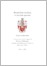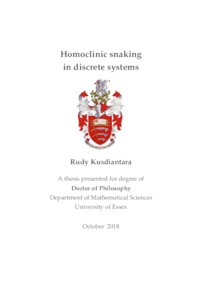# Homoclinic snaking in discrete systems

Kusdiantara, Rudy (2018) Homoclinic snaking in discrete systems. PhD thesis, University of Essex.Preview
Text
Kusdiantara_thesis.pdf

Download (17MB) | Preview

## Abstract

In this thesis, we investigate analytically and numerically bifurcations of localized solutions in discrete systems, i.e., the discrete Swift-Hohenberg, an optical cavity equation, and the discrete Allen-Cahn equation, which have infinitely multiplicity called homoclinic snaking. First, we study the discrete Swift-Hohenberg equation with cubic and quintic nonlinearity, obtained from discretizing the spatial derivatives of the Swift-Hohenberg equation using central finite differences. We investigate the discretization effect on the bifurcation behavior, where we identify three regions of the coupling parameter, i.e., strong, weak, and intermediate coupling. In the intermediate coupling region, multiple Maxwell points can occur for the periodic solutions and may cause irregular snaking and isolas. Theoretical analysis for the snaking and stability of the corresponding solutions is provided in the weak coupling region. Next, we study time-independent solutions of an optical cavity equation with saturable nonlinearity. When the nonlinearity is of Kerr-type (i.e., cubic), one obtains the discrete version of Lugiato-Lefever equation. The equation admits uniform and localized solutions. Localized solutions can be formed by combining two different uniform states, which can develop a snaking structure in their bifurcation diagram when a control parameter is varied, i.e., homoclinic snaking. C-shaped isolas may also occur when the background of localized states disappear at a certain bifurcation parameter value. The semi-analytical approximation is also proposed to determine the stability of the corresponding solutions. Finally, we present a study on time-independent solutions of the two-dimensional discrete Allen-Cahn equation with cubic and quintic nonlinearity. Three different types of lattices are considered, i.e., square, honeycomb, and triangular lattices. Localized solutions of discrete Allen-Cahn equation also can be formed by combining two different uniform states. We introduce an active-cell approximation, which is extended from the one-active site approximation in one-dimensional case for a weakly coupled system.

Item Type: Thesis (PhD) Q Science > QA Mathematics Faculty of Science and Health > Mathematical Sciences, Department of Rudy Kusdiantara 06 Nov 2018 15:15 06 Nov 2018 15:15 http://repository.essex.ac.uk/id/eprint/23314

### Actions (login required)View Item